{{announcement.body}}
{{announcement.title}}

# Logistic Regression Theory: An Overview

DZone 's Guide to

# Logistic Regression Theory: An Overview

### Get a detailed example of logistic regression theory and Sigmoid functions, followed by an in-depth video summarizing the topics.

· Big Data Zone ·
Free Resource

Comment (0)

Save
{{ articles.views | formatCount}} Views

Logistic Regression Theory | Quick KT

Logistic regression is used to predict the outcome of a categorical variable. A categorical variable is a variable that can take only specific and limited values.

Let's consider a scenario where we have data about some students. This data is about hours studied before an exam and whether they passed (yes/no or 1/0).

``````hoursStudied=[[1.0],[1.5],[2.0],[2.5],[3.0],[3.5],[3.6],[4.2],[4.5],[5.4],
[6.8],[6.9],[7.2],[7.4],[8.1],[8.2],[8.5],[9.4],[9.5],[10.2]]
passed =     [  0  ,0    ,  0  ,  0 , 0    ,0    ,  0  , 0   ,0    , 0   ,
1  , 0   , 0   , 1  ,   1  ,   1 , 1   ,   1 ,   1 ,   1 ]

print("  ",row,"    ----->",row)``````

Output:

``````hoursStudied  passed
1.0     -----> 0
1.5     -----> 0
2.0     -----> 0
2.5     -----> 0
3.0     -----> 0
3.5     -----> 0
3.6     -----> 0
4.2     -----> 0
4.5     -----> 0
5.4     -----> 0
6.8     -----> 1
6.9     -----> 0
7.2     -----> 0
7.4     -----> 1
8.1     -----> 1
8.2     -----> 1
8.5     -----> 1
9.4     -----> 1
9.5     -----> 1
10.2     -----> 1``````

Let's plot the data and see how it looks:

``````import matplotlib.pyplot as plt
%matplotlib inline

plt.ylabel("passed")``````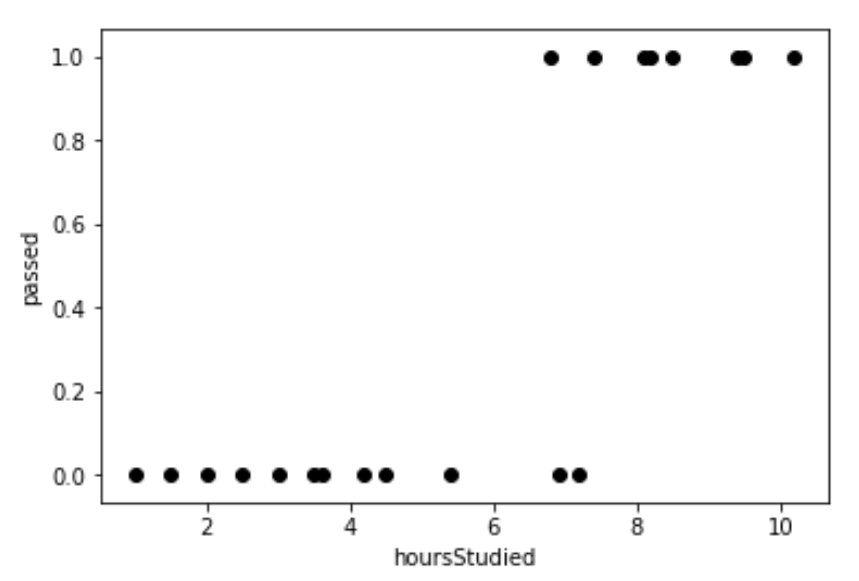If we plot a normal linear regression over our data points, it looks like this: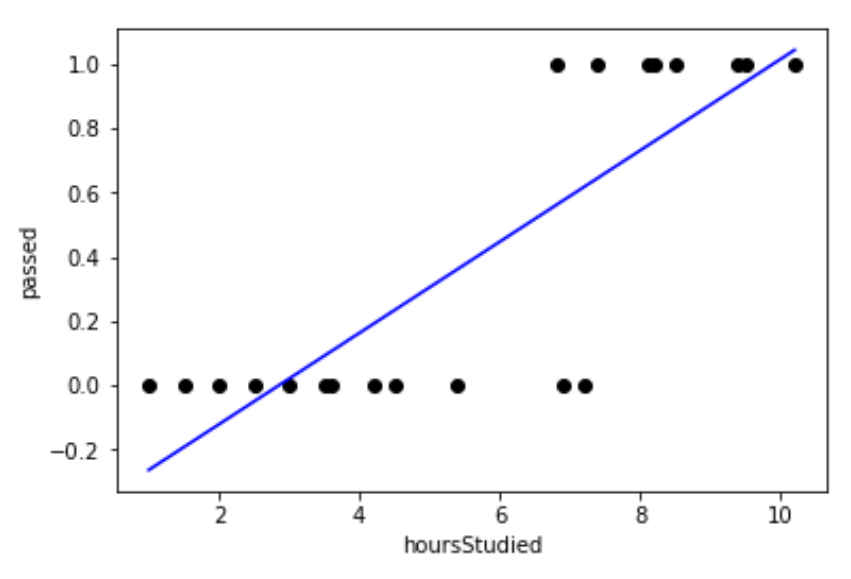We know that the output will be either 0 or 1. We can see that this regression produces all sorts of values between 0 and 1... but that's not the actual problem. It is also producing impossible values — negative values and values greater than one — which have no meaning.

We need a better regression line. Logistic regression is what we should use here. The logistic regression will fit our data points like this:Most often, we want to predict our outcomes as yes/no or 1/0. The logistic function is given by: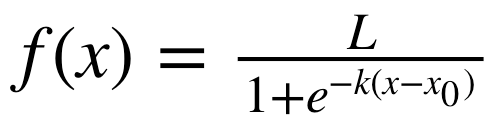...where:

• L = curve's maximum value

• k = steepness of the curve

• x0 = x value of Sigmoid's midpoint

Sigmoid's function is a standard logistic function (k=1,x0 = 0, L=1):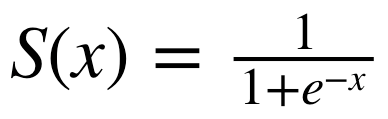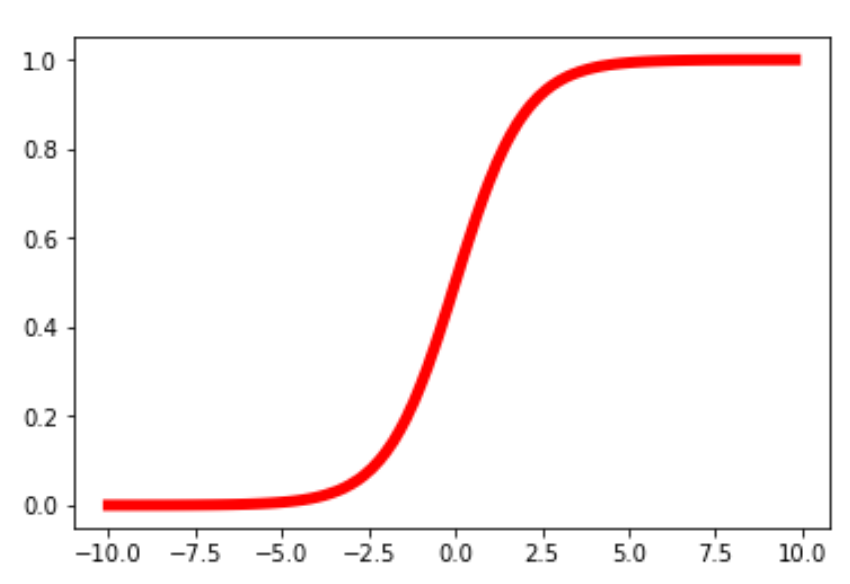The Sigmoid curve

The Sigmoid function has an S-shaped curve. It has a finite limit of 0 as x approaches negative infinity and 1 as x approaches positive infinity.

The output of the Sigmoid function when x=0 is 0.5. Thus, if the output is more than 0.5, we can classify the outcome as 1 (or yes) and if it less than 0.5, we can classify it as 0 (or no). For example, if the output is 0.65, we can say in terms of probability that there is a 65% chance that your favorite football team is going to win today.

Thus, the output of the Sigmoid function is not only able to be used to classify yes or no, it can also be used to determine the probability of yes/no.

Next, let's check how logistic/Sigmoid functions work in Python. We need math for writing the Sigmoid function and numpy to define the values for the X-axis, or matplotlib:

``````import math
import matplotlib.pyplot as plt
import numpy as np``````

Next, we'll define the Sigmoid function as described by the following equation: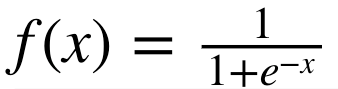``````def sigmoid(x):
a = []
for item in x:
#(the sigmoid function)
a.append(1/(1+math.exp(-item)))
return a``````

Now, we'll generate some values for x. It will have values from -10 to +10 with increments of 0.2.

``x = np.arange(-10., 10., 0.2)``

Output:

``````[-10.   -9.8  -9.6  -9.4
-9.2  -9.   -8.8  -8.6  -8.4
-8.2  -8.   -7.8  -7.6  -7.4
-7.2  -7.   -6.8  -6.6  -6.4
-6.2  -6.   -5.8  -5.6  -5.4
-5.2  -5.   -4.8  -4.6  -4.4
-4.2  -4.   -3.8  -3.6  -3.4
-3.2  -3.   -2.8  -2.6  -2.4
-2.2  -2.   -1.8  -1.6  -1.4
-1.2  -1.   -0.8  -0.6  -0.4
-0.2  -0.    0.2   0.4   0.6
0.8   1.    1.2   1.4   1.6
1.8   2.
2.2   2.4   2.6   2.8   3.
3.2   3.4   3.6   3.8   4.
4.2   4.4   4.6   4.8   5.
5.2   5.4   5.6   5.8   6.
6.2   6.4   6.6   6.8   7.
7.2   7.4   7.6   7.8   8.
8.2   8.4   8.6   8.8   9.
9.2   9.4   9.6   9.8]``````

We'll pass the values of x to our Sigmoid function and store its output variable in y:

``y = sigmoid(x)``

We'll plot the x values in the X-axis and the y values in the Y-axis to see the Sigmoid curve:

``````plt.plot(x,y)
plt.show()``````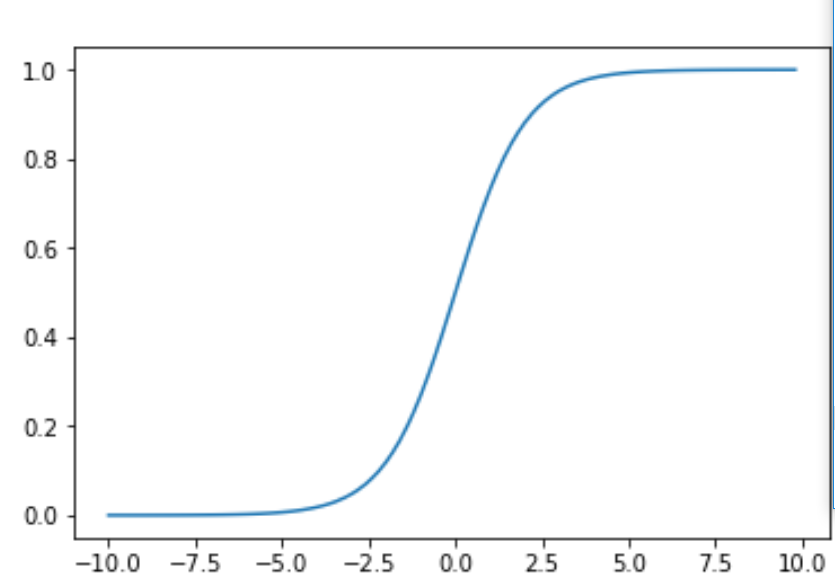We can observe that, if x is very negative, the output is almost 0. And if x is very positive, its output is almost 1. But when x is 0, y is 0.5.

Topics:
artificial intelligence ,big data ,data analytics ,data visualization ,deep learning ,logistic regression ,machine learning ,machine learning algorithm ,sigmoid function ,tutorial

Comment (0)

Save
{{ articles.views | formatCount}} Views

Published at DZone with permission of Vinay Kumar . See the original article here.

Opinions expressed by DZone contributors are their own.